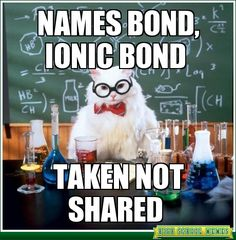# Ionic And Covalent Bonding

39 Questions | Attempts: 187
ShareSettings.

• 1.
Why is it necessary to use prefixes in naming covalent compounds?
• 2.
Name the following Covalent compound: SO3
• 3.
Name the following Covalent compound: P4O8
• 4.
Name the following Covalent compound: N­2O
• 5.
Write the formula for the covalent compound: Disulfur decaflouride
• 6.
Write the formula for the covalent compound: Phosphorous trichloride
• 7.
Write the formula for the covalent compound: Tetracarbon hexahydride
• 8.
Give the formula for the following Ionic compound: Calcium Iodide
• 9.
Give the formula for the following Ionic compound: Copper(II) chloride
• 10.
Give the formula for the following Ionic compound: Silver(I) Acetate
• 11.
Name the following Ionic compound: K2O
• 12.
Name the following Ionic compound: NaClO
• 13.
Name the following Ionic compound: KNO3
• 14.
Which is the charge that results when oxygen becomes an ion?
• A.

+2

• B.

-3

• C.

+3

• D.

-2

• 15.
Group 13 elements tend to acquire which charge when they form ions?
• A.

+3

• B.

-5

• C.

+5

• D.

-3

• 16.
A compound has the formula X3Y. For every 15 X atoms present in this compound, how many Y atoms are there?
• A.

3

• B.

5

• C.

45

• D.

15

• 17.
Which is a physical property of ionic compounds in their solid state?
• A.

Good conductor of electricity

• B.

Weak attractive forces between ions

• C.

Low boiling point

• D.

High melting point

• 18.
Which is the correct formula for the compound formed between beryllium and nitrogen?
• A.

BeN

• B.

Be3N

• C.

Be3N2

• D.

Be2N3

• 19.
What is the correct formula for the compound formed between magnesium and the phosphate ion?
• A.

MgPO4

• B.

Mg3PO2

• C.

Mg3(PO4)2

• D.

MgP2O8

• 20.
Which is the correct formula for the compound Manganese (III) Fluoride?
• A.

MnF

• B.

Mn3F

• C.

MnF3

• D.

Mn3F3

• 21.
Which is the correct formula for the compound Chromium (II) Nitrate?
• A.

(Cr)2NO3

• B.

Cr2NO3

• C.

CrNO2

• D.

Cr(NO3)2

• 22.
How many single covalent bonds are elements in Group 16, light blue, likely to form?
• A.

1

• B.

2

• C.

3

• D.

4

• 23.
Which combination of bonds is present in this molecule?
• A.

Single Bond

• B.

Double Bond

• C.

Triple Bond

• D.

• 24.
Which type of molecular shape is shown by this molecule?
• A.

Trigonal pyramidal

• B.

Tetrahedral

• C.

Bent

• D.

Trigonal planar

• 25.
Which one do you like?
• A.

Trigonal pyramidal

• B.

Tetrahedral

• C.

Bent

• D.

Trigonal planar

## Related TopicsBack to top
×

Wait!
Here's an interesting quiz for you.